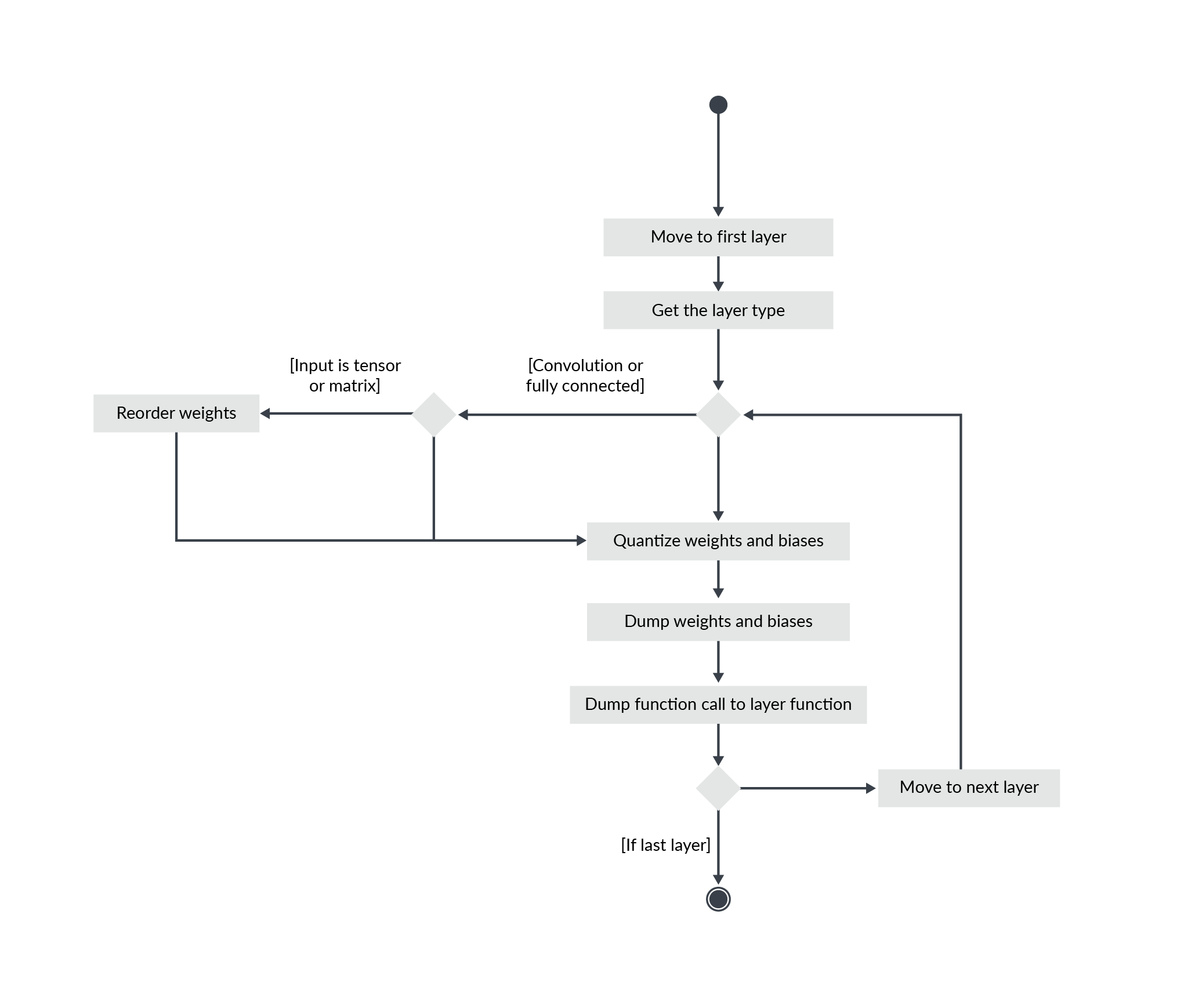## Generate the CMSIS-NN implementation

Once the Q-formats are known and the bias and out shifts are known, you are ready to generate the quantized coefficients and code for use with CMSIS-NN.

Start with the first layer. If it is a fully connected or convolutional layer, quantize the reordered weights and quantize the biases. Then dump those coefficients into C arrays. Dump a function call to the layer function using the bias, and the out shift which were computed for this layer and the parameters of the layer.

If it is another kind of layer, just dump a function call to the layer function using the correct parameters for the layers.

Parameters include, for example, stride, padding, kernel size, input dimensions, and output dimensions.

The process is summarized in the following diagram:Each layer requires several buffers for its processing:

• Input and output buffers, which can be the same if the layer is doing in place modifications
• Temporary buffers for q7 versions of some functions

All of those buffers must also be allocated when generating the C code.

Finally, you need to look at the input and output of the network.

The input has no reason to be in the Q-format expected by the network. The input is in the format defined by your sensors and your pre-processing code.

The input buffer of the network should be initialized with values in the right Q-format. This means that some pre-processing may have to be added if the input is generated on the device in a format which is not adapted.

If the format conversion of the input adds too much overhead for the application, then the input Q-format may be chosen differently to avoid this pre-processing step. In that case, this constraint should be considered when computing the Q-formats for all the layers.

This different format may not be the one giving the best result, so testing is required, as explained in the Testing this result section of this guide.

The output may have to be converted, too, if the code following the network is expecting values with a different format than the format generated by the network. If the latest layer of the network is not tunable, because there is no shift parameter, then a post-processing step may be required to do this conversion.